Chapter Notes: Statistics

# Statistics Chapter Notes - Mathematics (Maths) Class 10

 Table of contentsMeanMedianModeCumulative Frequency ChartEmpirical Formula between Mode, Mean and Median## Mean

The mean value of a variable is defined as the sum of all the values of the variable divided by the number of values.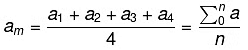## Median

The median of a set of data values is the middle value of the data set when it has been arranged in ascending order.  That is, from the smallest value to the highest value
Median is calculated as: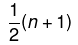Where n is the number of values in the data.
If the number of values in the data set is even, then the median is the average of the two middle-value.

## Mode

Mode of statistical data is the value of that variable that has the maximum frequency.

### Mean for Ungroup Frequency Table

Here is the ungroup frequency table: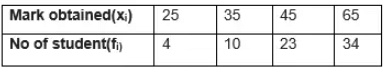Mean is given by: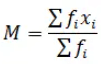Greek letter ∑ (capital sigma) means summation.

### Mean for Group Frequency Table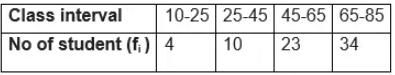In this distribution, it is assumed that the frequency of each class interval is centered around its mid-point i.e class marks.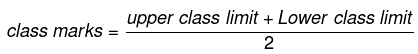### Mean can be calculated using three methods:

(a) Direct Method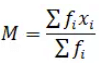This method can be very calculation-intensive if the values of f and x are large. We have big calculations and chance of making mistake is quite high

Steps involved in finding the mean using Direct Method

• Prepare a frequency table with the help of class marks
• Multiply fi and  xand find the sum of it.
• Use the above formula and find the mean.

Example: The following table shows the weights of 10 children: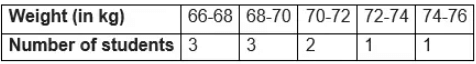Find the mean by using the direct method.
Sol: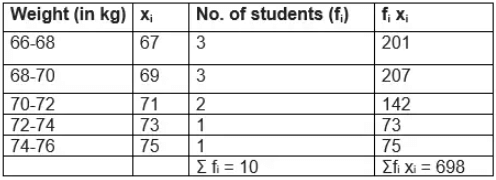So, Mean would be
=698/10  = 69.8 kg.

(b) Assumed Mean Method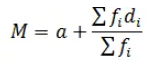Where
a= Assumed Mean
di = xi –a

This method is quite useful when the values of f and x are large. It makes the calculation easier. In this method, we take some assumed mean calculate the deviation from it and then calculate the mean using the above formula.

Steps involved in finding the mean using the Assumed Mean Method

• Prepare a frequency table.
• Choose A and take deviations ui = (xi -a)/h of the values of xi .
• Multiply fi ui and find the sum of it.
• Use the above formula and find the mean.

Example: The following table shows the weights of 10 children: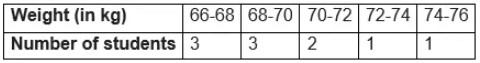Find the mean by using Assumed Mean method.
Sol:
Let the assumed mean = A = 71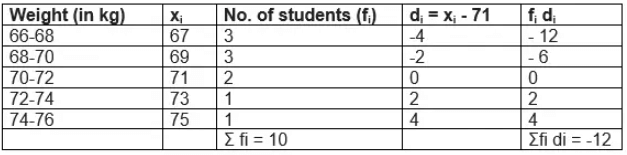So, Mean would be
= 71-12/10  = 69.8 kg
c) Step Deviation Method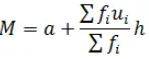Where
a= Assumed mean
ui  = (xi –a)/h
This method is quite useful when the values of f and x are large. It makes the calculation further easier by dividing the deviation from the common factor.

Steps involved in finding the mean using Step Deviation Method

• Prepare a frequency table.
• Choose A and h and take ui = (xi –a)/h of the values of xi .
• Multiply fi u i and find the sum of it.
• Use the above formula and find the mean.

Example: The following table shows the weights of 10 children: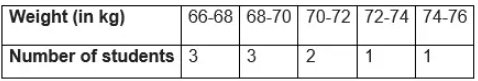Find the mean by using Step Deviation method.
Sol:

Let the assumed mean = A = 71  and h=2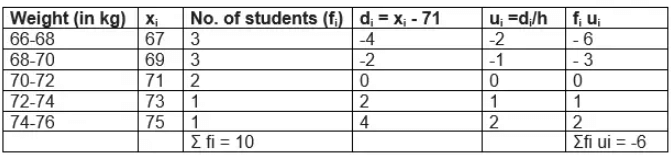So, Mean would be
=71+ (-6/10) 2  = 69.8 kg

### Mode for Grouped Frequency Table

Modal Class: The class interval having highest frequency is called the modal class and Mode is obtained using the modal class
Mode formula is given as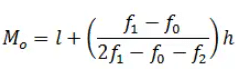Where
l = lower limit of the modal class,
h = size of the class interval (assuming all class sizes to be equal),
f1 = frequency of the modal class,
f0 = frequency of the class preceding the modal class,
f2 = frequency of the class succeeding the modal class.
Example: The following table shows the ages of the patients admitted in a hospital during a year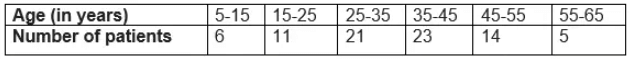Find the mode.
Sol:
Modal class = 35 – 45, l = 35, class width (h) = 10, f1 = 23, f0 = 21 and f2 = 14
Substituting the values in the Mode formula given above we get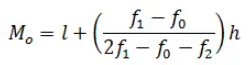Mode= 36.8 year

## Cumulative Frequency Chart

The cumulative frequency of a class is the frequency obtained by adding the frequencies of all the classes preceding the given class.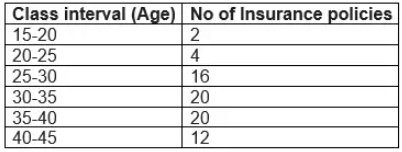Cumulative Frequency chart will be like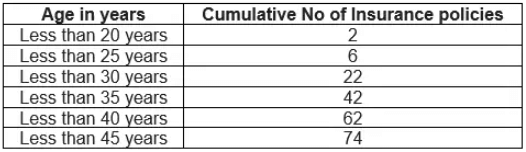The above table cumulative frequency distribution of the less than type. We can similary make it like below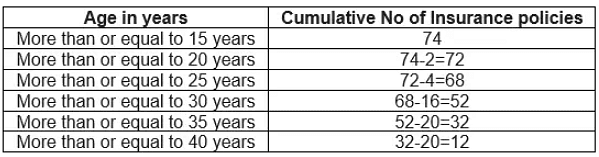The table above is called a cumulative frequency distribution of the more than type.

### Median of a Grouped Data Frequency Table

How to find Median of a grouped data frequency table

• For the given data, we need to have class interval, frequency distribution and cumulative frequency distribution
• Then we need to find the median class
How to find the median class
(a) we find the cumulative frequencies of all the classes and n/2
(b)We now locate the class whose cumulative frequency is greater than (and nearest to) n/2
(c)That class is called the median class
• Median is calculated as per the below formula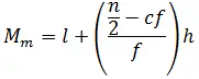Where
l = lower limit of median class,
n = number of observations,
cf = cumulative frequency of class preceding the median class,
f = frequency of median class,
h = class size (assuming class size to be equal)

Example: A survey regarding the heights (in cm) of 60 girls  of a school was conducted and the following data was obtained: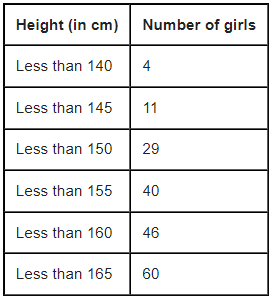Find the median height.

Sol:
To calculate the median height, we need to find the class intervals and their corresponding frequencies.
The given distribution being of the less than type, 140, 145, 150, . . ., 165 given the upper limits of the corresponding class intervals. So, the classes should be below 140, 140 - 145, 145 - 150, . . ., 160 - 165. Observe that from the given distribution, we find that there are 4 girls with height less than 140, i.e., the frequency of class interval below 140 is 4 . Now, there are 11 girls with heights less than 145 and 4 girls with height less than 140. Therefore, the number of girls with height in the interval 140-145 will be 11-4=7. Similarly, other can be calculated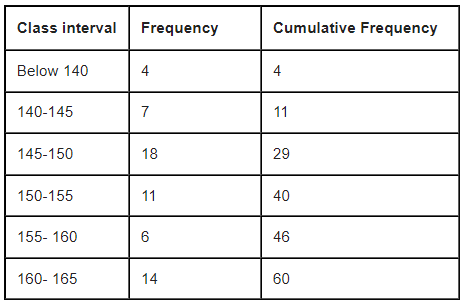So, n =60 and n/2=30 And cumulative frequency which is greater than and nearest to 30 is 40 , So median class 150-155
l (the lower limit) = 150,
cf (the cumulative frequency of the class preceding 150 - 155) = 29,
f (the frequency of the median class 150 - 151) = 11,
h (the class size) = 5.
Now by Median Formula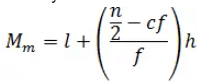= 150 + [(30-29)/11]5
=150.45 cm

## Empirical Formula between Mode, Mean and Median

Empirical Formula between Mode, Mean and Median is given as 3 Median=Mode +2 Mean

### Graphical Representation of Cummulative Frequency Distribution

We can represent Cummulative frequency distribution on the graph also. To represent the data in the table graphically, we mark the upper limits of the class intervals on the horizontal axis (x-axis) and their corresponding cumulative frequencies on the vertical axis (y-axis), choosing a convenient scale.

When we draw the graph for the cumulative frequency distribution of the less than type. The curve we get is called a cumulative frequency curve, or an ogive (of the less than type).

When we draw the graph for the cumulative frequency distribution of the more than type. The curve we get is called a cumulative frequency curve, or an ogive (of the more than type).

When we plot both these curve on the same axis, The two ogives will intersect each other at a point. From this point, if we draw a perpendicular on the x-axis, the point at which it cuts the x-axis gives us the median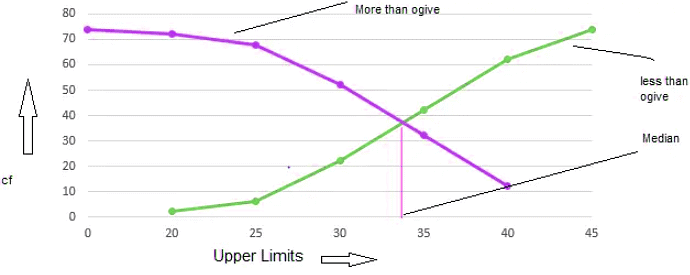The document Statistics Chapter Notes | Mathematics (Maths) Class 10 is a part of the Class 10 Course Mathematics (Maths) Class 10.
All you need of Class 10 at this link: Class 10

## Mathematics (Maths) Class 10

115 videos|478 docs|129 tests

## FAQs on Statistics Chapter Notes - Mathematics (Maths) Class 10

 1. What is the formula to calculate the mean?Ans. The formula to calculate the mean is: Mean = Sum of all values / Total number of values
 2. How do you calculate the mode for grouped data?Ans. To calculate the mode for grouped data, we find the class interval with the highest frequency. Within that interval, we calculate the modal group by subtracting the frequency of the previous interval from the frequency of the current interval, and dividing it by the difference between the frequency of the current interval and the frequency of the next interval. Finally, we calculate the mode using the formula: Mode = Lower limit of modal group + (Modal group frequency - Frequency of previous interval) * (Class width / Difference between modal group frequency and frequency of next interval)
 3. What is the cumulative frequency?Ans. Cumulative frequency is the accumulation of the frequencies of all the previous data points or class intervals up to a specific point. It helps in analyzing the total frequency distribution and finding the median, quartiles, and percentiles.
 4. How do you calculate the median for grouped data?Ans. To calculate the median for grouped data, we first find the cumulative frequency. Then, we identify the class interval that contains the median value. Using the formula: Median = Lower limit of median class + ((Total frequency / 2) - Cumulative frequency of previous class) * (Class width / Frequency of median class)
 5. What is the empirical formula that relates mode, mean, and median?Ans. The empirical formula that relates mode, mean, and median is: 3 * Mean = Mode + 2 * Median

## Mathematics (Maths) Class 10

115 videos|478 docs|129 testsExplore Courses for Class 10 examSignup to see your scores go up within 7 days! Learn & Practice with 1000+ FREE Notes, Videos & Tests.
10M+ students study on EduRev
Track your progress, build streaks, highlight & save important lessons and more!
Related Searches

,

,

,

,

,

,

,

,

,

,

,

,

,

,

,

,

,

,

,

,

,

;